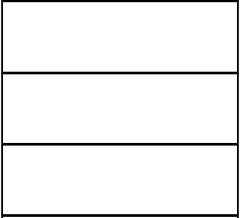# A yard

Yevgen is fencing in a yard that is 30 meters longer than it is wide. The yard will have an area of 1000 m2. Find its width and length.

y =  50 m
x =  20 m

### Step-by-step explanation:Did you find an error or inaccuracy? Feel free to write us. Thank you!

Tips for related online calculators
Are you looking for help with calculating roots of a quadratic equation?
Do you have a linear equation or system of equations and looking for its solution? Or do you have a quadratic equation?

#### Grade of the word problem:

We encourage you to watch this tutorial video on this math problem: